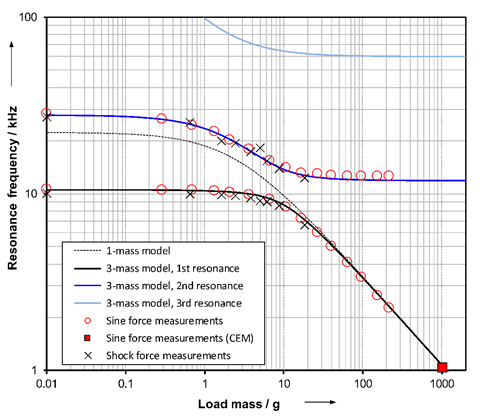# Case study on model-based dynamic calibration of a small strain gauge force transducer

12.09.2017

Investigations of the model-based dynamic calibration of a small strain gauge force transducer of high bandwidth revealed new challenges for parameter identification. A more generalized mechanical model now assumes the force transducer to be elastically connected to the measurement set-up at both ends. Based on new experimental sine and shock force data, the model was studied and the parameters of the force transducer were identified. The proposed model can link the calibration results of different calibration methods (shock and sinusoidal excitation) to a much better degree than before.

When performing a model-based calibration, the dynamic behavior of a force transducer is determined by means of a model where the force transducer and the calibration device are described by a series arrangement of spring-mass-damper elements. The sought stiffness and damping parameters of the force transducer and its internal masses are to be identified from the measurement data. The purpose of the model-based calibration is the general characterization of the transducer’s dynamic behavior independent of the given measurement application or its type of dynamic force excitation. Calibrations with shock forces or sinusoidal forces must therefore give consistent model parameters.

Initial investigations of a small strain gauge force transducer of high bandwidth demonstrated discrepancies that could now be explained by means of an enlarged model and new extensive measurements. Here, the force transducer HBM U9B/1 kN, examined as a case study, was tested at the 20 kN shock force calibration device (see Figure 1) of Working Group 1.73 “Impact Dynamics” and at the high-frequency acceleration standard measuring device (see Figure 2) of Working Group 1.71 “Realization of Acceleration” by applying shock and sinusoidal excitations. Additional load masses of different size allowed a defined variation of the dynamic behavior. By comparing the experimentally measured and the modelled resonance frequencies, the model was fitted and the stiffness parameters of the force transducer were identified. Additional investigations with finite element methods confirm the experimental results obtained with the different measurement set-ups.Figure 1: Shock force tests at the 20 kN shock force calibration device using a small
pendulum (7 g) and additional load masses; the photo shows the force transducer with a
load mass of about 19 g made of brass mounted at the airborne reaction mass of 10 kg.Figure 2: Investigation of the force transducer with different load masses applying sinusoidal
excitation up to 30 kHz at the high-frequency acceleration standard measuring device.

The investigated force transducer exhibits two dominant resonances along its measuring axis in the frequency range of interest, which develop differently depending on the size of the load mass.  For very small values (e.g. shock calibration without load mass), the lowest resonance is caused by the coupling resonance of the transducer housing, and for large values, by the elastically coupled load mass. The force transducer has threaded rods at both ends, and their coupling stiffness has an influence on the dynamic behavior which must not be neglected.

A more generalized model now considers the force transducer to be elastically connected at both ends. In the past, the connection at the base was assumed to be rigid, and only the measuring spring including the load mass was assumed to be elastic. The shock as well as the sine force calibration are clearly described by a model of three elastically coupled model masses (reaction mass, base part of the transducer housing, upper part of transducer housing including the load mass). Figure 3 presents the experimental and theoretical values of the resonance frequencies described by this model in dependence of the applied load mass. The model parameters of the force transducer were identified by sine excitation. The diagram additionally displays the resonance frequencies obtained by shock excitation. The measurement value for a load mass of 1 kg (measured at the Spanish metrology institute CEM) demonstrates a typical sine force calibration with masses in the kilogram range and excitations up to a few kilohertz. The second resonance could not be detected and the consequently derived dynamic behavior (using a 1-mass model) would give drastic deviations for small load masses. This previously observed discrepancy is explained by the new model. Shock and sine force calibration yield consistent model parameters of the force transducer. The dynamic measurement behavior described by the model-based calibration may now be transferred to a given application using an appropriate model extension.Figure 3: Comparison of measured and modelled resonance frequencies of the force transducer with different load masses.

The work on modelling, data analysis and parameter identification was performed in close cooperation with Working Group 8.42 “Data Analysis and Measurement Uncertainty”. Further information on the case study presented can be found in .

### Literature:

 M. Kobusch, S. Eichstädt, “A case study in model-based dynamic calibration of small strain gauge force transducers”, ACTA IMEKO, ISSN: 2221-870X, April 2017, Vol. 6, No. 1, 3-12. DOI:10.21014/acta_imeko.v6i1.433

### Contact person:

Michael Kobusch, FB 1. 7, AG 1. 73, E-Mail:michael.kobusch(at)ptb.de